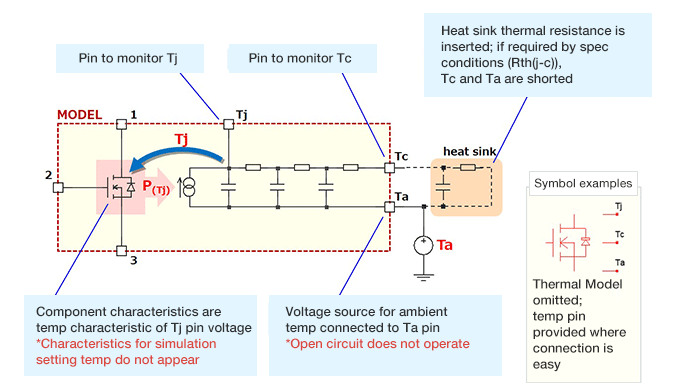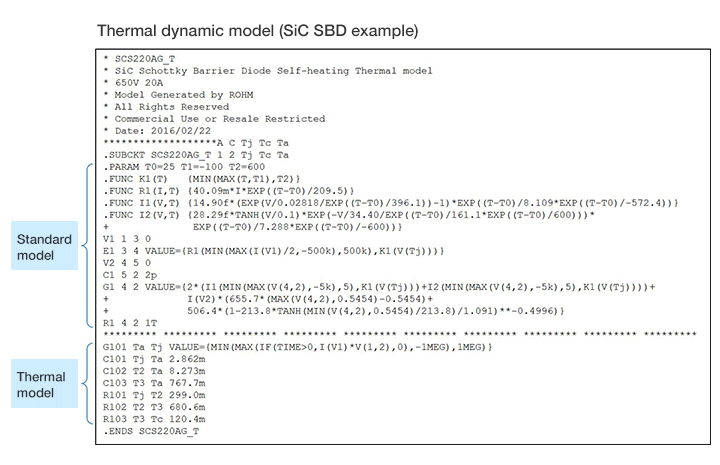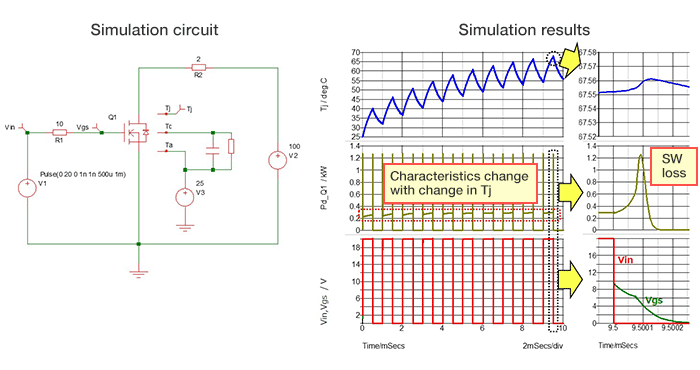### Simulation

2020.08.19

・The Thermal Dynamic Model is a SPICE model that incorporates the Thermal Model and represents changes in characteristics due to heat generation of the component itself.

・The Thermal Dynamic Model is a model in which the Thermal Model is added to the subcircuit for a device.

・The incorporated Thermal Model is used to calculate Tj, and the Tj is reflected in the electrical characteristics of the device.

In the previous article we explained in summary the Thermal Model, which is a SPICE model used in simulations relating to heat. In this article, we give a brief explanation of a Thermal Dynamic Model, which is another SPICE model used in thermal simulations.

## About the Thermal Dynamic Model

The Thermal Dynamic Model is a SPICE model that incorporates the Thermal Model explained previously, and represents changes in characteristics due to the heat generation of components themselves. The losses of a component and the Thermal Model incorporated in the Thermal Dynamic Model are used to compute Tj, and the result for Tj is reflected in the electrical characteristics of the component.The following is an example of source code in the Thermal Dynamic Model. The component is a SiC Schottky barrier diode (SCS220), but the approach is the same as that in the diagram above, with the Thermal Model added to the subcircuit model for the SiC SBD.An example of use of a Thermal Dynamic Model is shown below. The circuit should be thought of as the schematic diagram presented initially. Q1 is a SiC MOSFET, a heat sink is provided, and Ta = 25°C is given as a condition (25 V DC is applied to the Ta pin). From the simulation results it is seen that, due to MOSFET switching, Tj rises (as in the upper graph), and the change in Tj results in a change in the characteristics of Q1 (middle, lower graphs).